# Chapter 14 Estimating Population Characteristics - Part II: Plausible Values

In this session, we will learn about
- What Plausible Values are.
- Why Plausible Values are needed.
- How Plausible Values are produced and used.

## 14.1 Introduction

Plausible values have become a key statistical term since the introduction of international large-scale assessments. The data releases of PISA and TIMSS include plausible values as student ability measures. Most secondary data analyses use plausible values. What are plausible values, or, PV, for short, and how are plausible values used? In this chapter, we will explain the concepts of PV and procedures of using PV in secondary data analyses.

## 14.2 What are plausible values?

In Section 13.11, we explain the term “posterior distribution.” In that example, the posterior distribution is the probability distribution of a student’s likely ability, given the student’s test score, or item responses. For example, Table 13.4 shows that if a student obtained a score of 5 out of 10, then there is 0.01 probability the student’s ability is 0.1, 0.51 probability the student’s ability is 0.3, 0.38 probability the ability is 0.5, and 0.10 probability the ability is 0.7. That is, instead of providing a point estimate of the student’s ability, we provide a probability distribution of the likely abilities. Plausible values are random draws from the posterior distribution. So if one PV is drawn for a student, it is one possible ability measure for the student. If 1000 PV’s are drawn for a student, the sampling distribution of the 1000 PVs is an approximation to the posterior distribution. The following R code shows how we can sample 10000 observations from the posterior distribution for the test score of 5:

sample_ability <- sample(c(0.1,0.3,0.5,0.7,0.9),10000,replace=TRUE,prob=c(0.01234568, 0.50617284, 0.38271605, 0.09876543, 0.00000000))
table(sample_ability)
## sample_ability
##  0.1  0.3  0.5  0.7
##  140 5071 3766 1023
hist(sample_ability, main="Histogram of sampled abilities")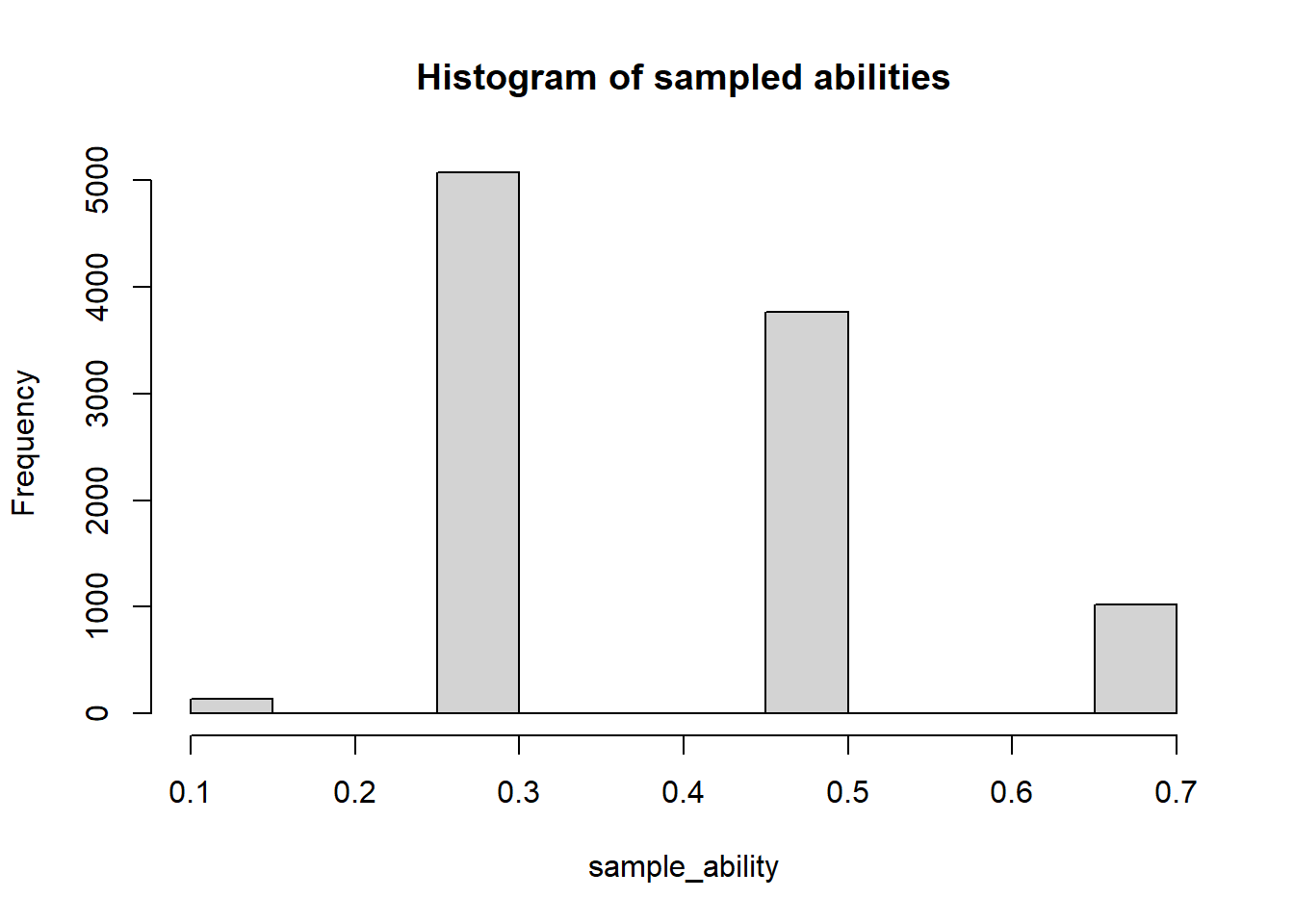Figure 14.1: Sample from Posterior Distribution

The 10000 random draws from the posterior distribution can be regarded as 10000 plausible values, and the collection of the 10000 PVs is the sampling distribution of the posterior distribution, as shown in the histogram above.

In summary, plausible values reflect a student’s likely ability measures, representing a probability distribution rather than a point estimate. In large-scale assessments, typically, 5 or 10 PVs are drawn for each student. With such small numbers of PVs, the PV’s will not represent the posterior distribution for each student very well. But when we aggregate the PV’s across students, we still get a good estimate for population statistics such as the mean and the variance.

## 14.3 Graphical Display of Prior, Posterior Distributions and Plausible Values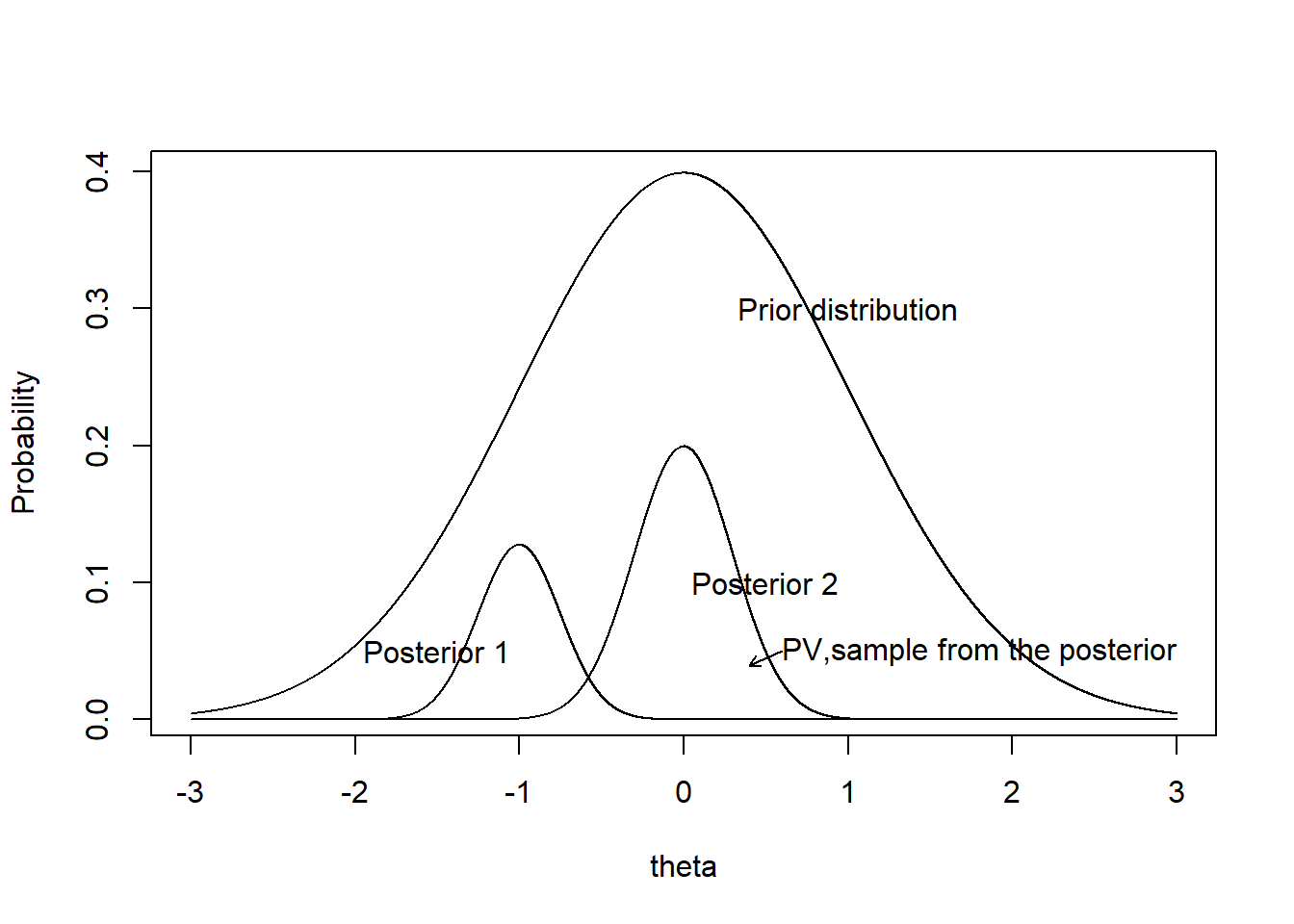Figure 14.2: Prior and Posterior Distributions

Prior Distribution
This is the student ability distribution. It is the distribution we want to estimate, so we can describe the student abilities in a population. For example, the mean of student abilities is 1.3 in Country X, and the variance is 1.6.

Posterior Distribution
For each student, there is a posterior distribution derived from the prior and the item responses, as shown in Section 13.11. Note that the posterior is narrower than the prior. This is because we have additional information from the item responses to narrow down the possible ability range of a student. If we do not have item responses, then the likely ability estimate for any student is just a random draw from the prior. But once we gather more information about a student through their item responses, we can “home-in” to where the student is located on the ability scale. The longer a test is, the narrower the posterior distribution, reflecting more precision with which we estimate a student’s ability. Without any item responses, the posterior distribution for any student is just the prior.

Further, the sum of the posterior distributions for all the students will make up the prior.

Plausible Values
Plausible values are sample observations from the posterior distribution. If many plausible values are drawn, then the histogram of the plausible values will be close to the posterior distribution. Each plausible value can be viewed as one possible ability measure for a student.

## 14.4 Why do we need Plausible Values?

In the MML estimation process, the mean and the variance of the prior distribution, in addition to the item parameters, are estimated. That is, after MML estimation, we already have an estimate of the population distribution. This should be sufficient for us, without worrying about computing posterior distributions for each student or drawing plausible values.

But in international large-scale assessments, we are always provided with individual student’s plausible values. There are at least two reasons for this.

First, while international assessment organisations provide estimated country mean and variance scores and percentile points of the ability distribution for each participating country, the results may not be detailed enough for some policy makers in each country. Within each country, there are many subgroups of students such as rural versus urban, ethnic groups, and many other groups of interest to inform policy. In addition, there are also research questions specific to the country that require tailored data analysis. To carry out these analyses, the countries need to have expertise to carry out IRT scaling such as the MML estimation and other complex procedures. As some countries do not have expertise in psychometric analysis, the aim is to provide countries with data sets that can be analysed using familiar software programs such as SPSS or SAS, rather than specialised IRT software programs.

Second, for the computation of standard errors of estimates, the sampling design needs to be taken into account and a replication/bootstrap technique is used where population estimates are repeatedly computed with slightly difference sets of individual ability measures.

For both of these reasons, individual student ability measures are required. However, we showed in Section 13.6 that individual ability estimates such as the WLE produce biased results when aggregated. Plausible values can overcome these issues, as we shall see in the sections below.

## 14.5 Producing plausible values

In the above example, we assumed a discrete distribution for the prior where there are only five ability groups. This was for the purpose of illustration of the concept of MML estimation method. When we actually carry out an MML estimation, the prior is typically assumed to be normally distributed. The mean and the variance of the normal distribution are estimated from the item responses.

We will demonstrate how plausible values are produced using the TAM package. We will use simulation to generate the item response data. The following R code generates a data set, analyses it with the MML estimation method, and produces some basic results. In addition, plausible values are drawn.

library(TAM)
generateRasch <- function(N,I){
theta <- rnorm( N ) # student abilities from normal distribution with mean 0 and var 1.
p1 <- plogis( outer( theta , seq( -2 , 2 , len=I ) , "-" ) )  #item diff from -2 to 2
resp <- 1 * ( p1 > matrix( runif( N*I ) , nrow=N , ncol=I ) )  # item responses
colnames(resp) <- paste("I" , 1:I, sep="")
return(list(resp=resp,theta=theta))
}
#Generate Rasch item responses for 10000 students and 30 items
generateData <- generateRasch(10000,30)
resp <- generateData$resp theta <- generateData$theta

#Fit item response data to the Rasch model and estimate student abilities
mod1 <- tam.mml(resp)

mod1$xsi #item difficulty parameters mod1$beta #population mean score (for the prior)
mod1$variance #population variance *for the prior) pv <- tam.pv(mod1) #Draw plausible values. By default, 10 PVs are drawn for each student. mean(pv$pv$PV1.Dim1) #Take every student's first PV, and calculate the mean var(pv$pv$PV1.Dim1) #Take every student's first PV, and calculate the variance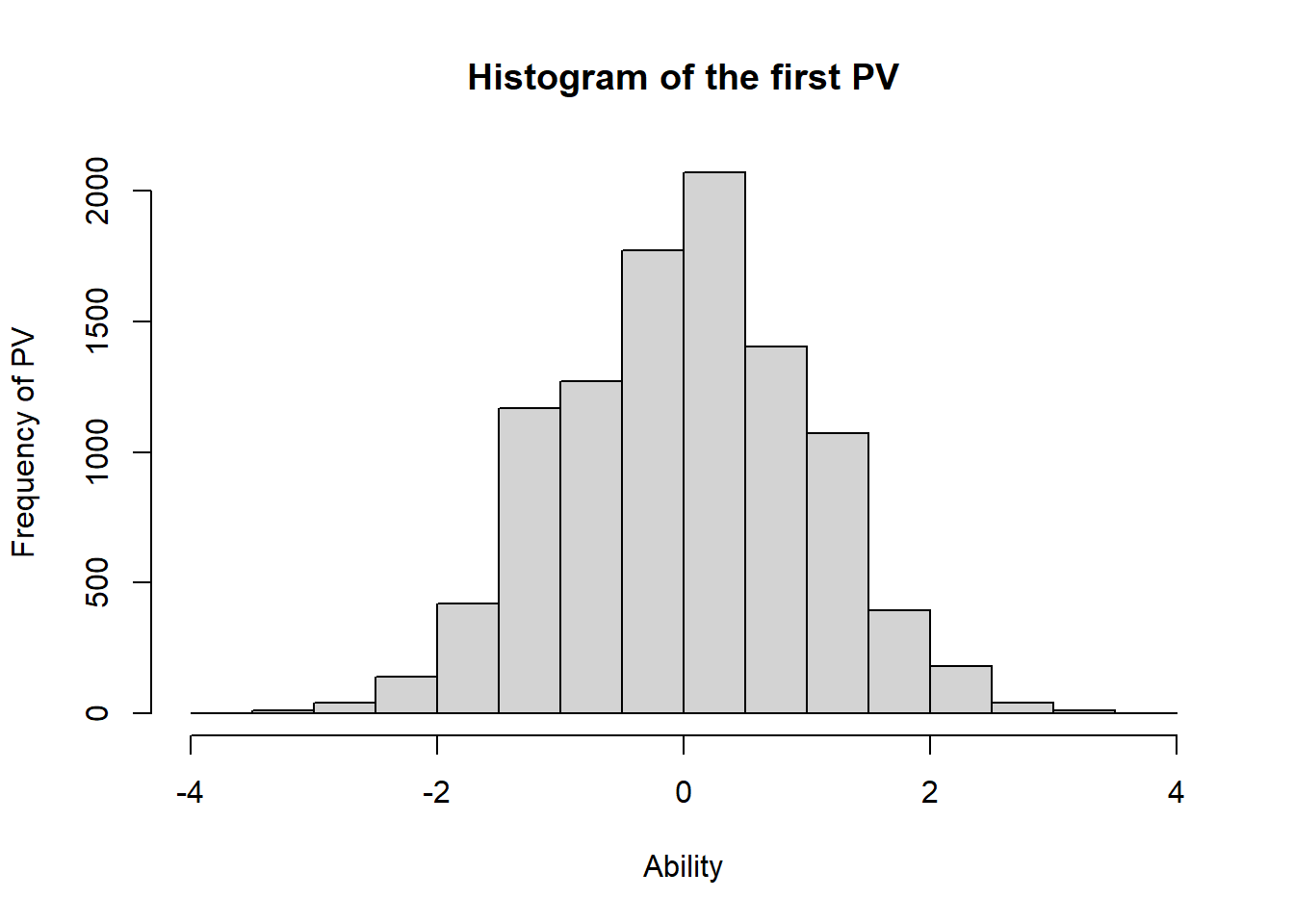Figure 14.3: Histogram of the first PV Both the directly estimated variance “mod1$variance” and the variance of the plausible values “var(pv$pv$PV1.Dim1)” are close to 1, the generating value.

## 14.6 Exercise 1

Re-run the above R code using the second PV, third PV, etc. Do the results differ much?

Re-run the above R code with different numbers of items, in particular, for short tests like 10 items. When tests are short, the measurement error is large, and the bias in variance estimate using WLE is also large. How does the MML estimation perform regarding very short tests?

## 14.7 Do NOT average multiple PVs first for each student

One important note to make is that the 10 PVs must NOT be averaged first, before computing population mean and variance score. Below we will see the effect of averaging the PVs for each student.

Run the following R code, assuming that PVs have been drawn.

pv10 <- pv$pv[,-1] #Remove 1st column which is person ID. pv_ave <- apply(pv10,1,mean) #Calculate mean across 10 PVs for each student mean(pv_ave) var(pv_ave) This time, the variance of averaged PVs is under 1. It is an under-estimate of the generating variance. ## 14.8 Expected a Posteriori (EAP) - The Average of PVs for Each Student When we average the PVs for each student, we are essentially computing the mean of the posterior distribution, since plausible values are random draws from the posterior distribution. The mean of the posterior distribution is called Expected A Posteriori (EAP) estimate. Sometimes people have used this as the ability estimate for a student. We do not recommend using EAP. If you want to assign a point estimate (a single number) for each student, it will be better to use the Weighted Likelihood Estimate (WLE). In addition, the use of EAP estimate to form population statistics will likely have bias, as shown in the above section. ## 14.9 Why plausible values are better than WLE and EAP for population estimates We have shown so far that using plausible values as student ability measures to estimate group variance produced no bias, while WLE over-estimates the variance and EAP under-estimates the variance. In addition to the unbiased estimation of group variance, plausible values also reconstruct a continuous ability distribution, while WLE and EAP have discrete distributions. The following shows the histograms of WLE, EAP and PV respectively for a 10-item test with 10000 students.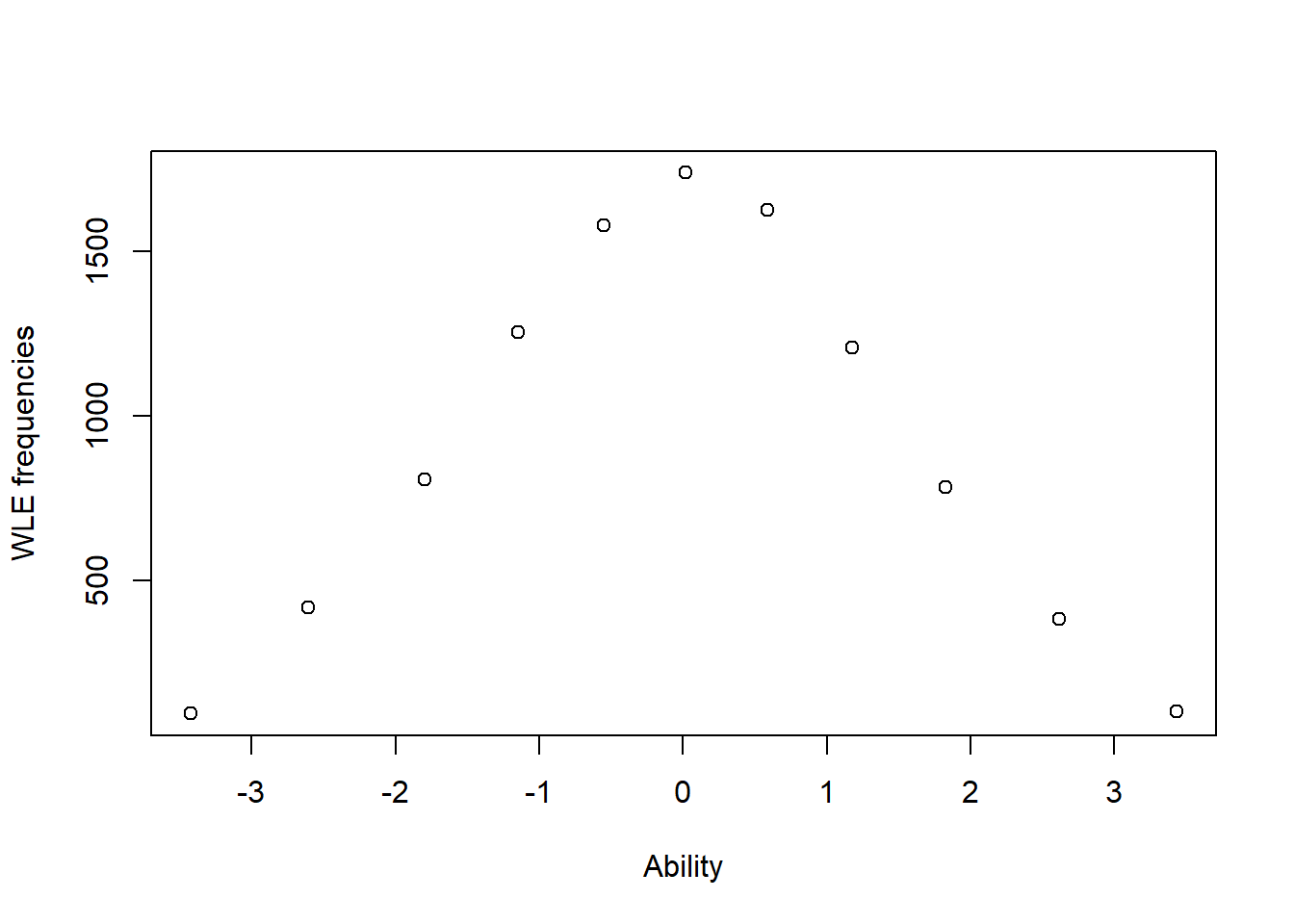Figure 14.4: WLE ability distribution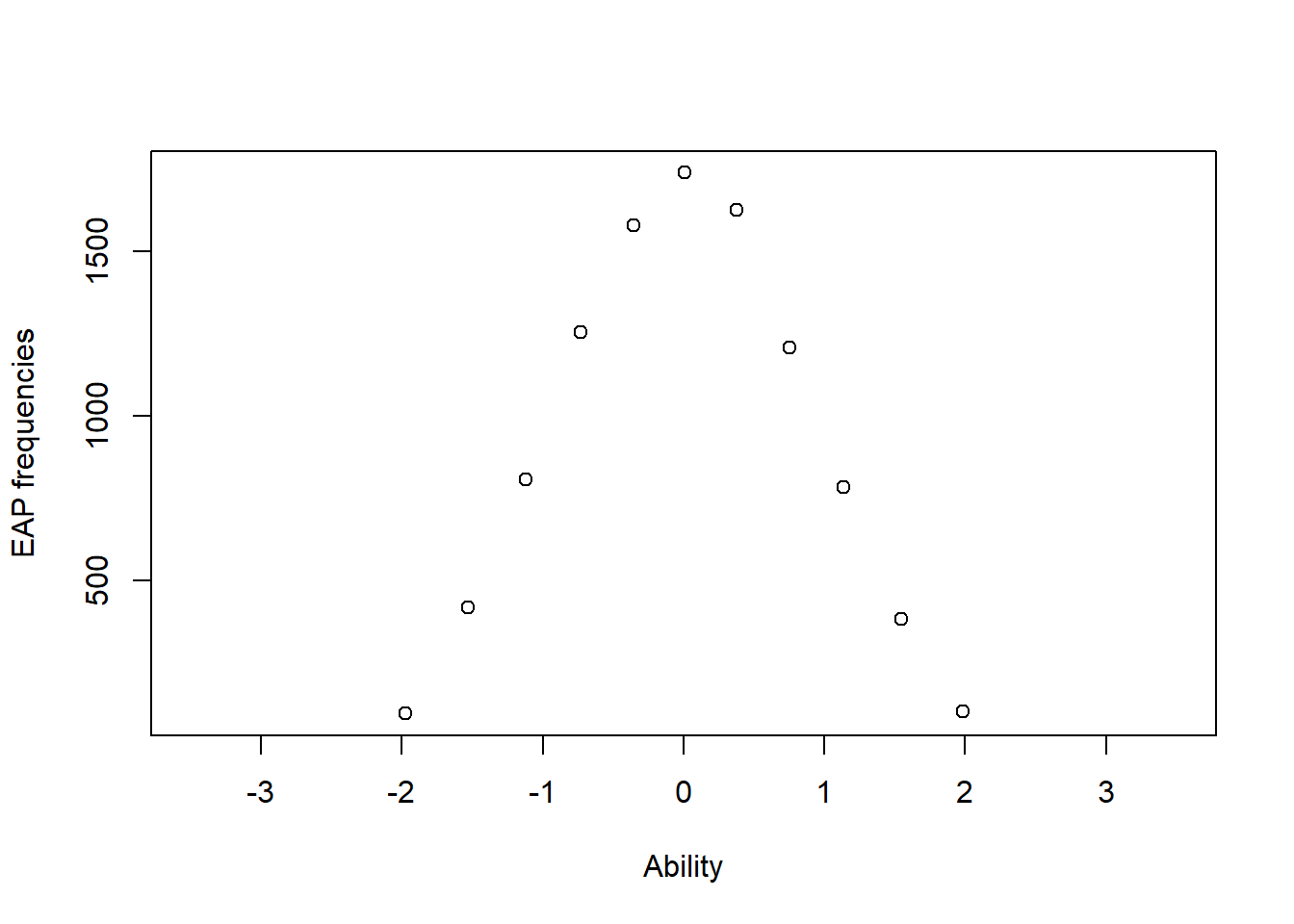Figure 14.5: EAP ability distribution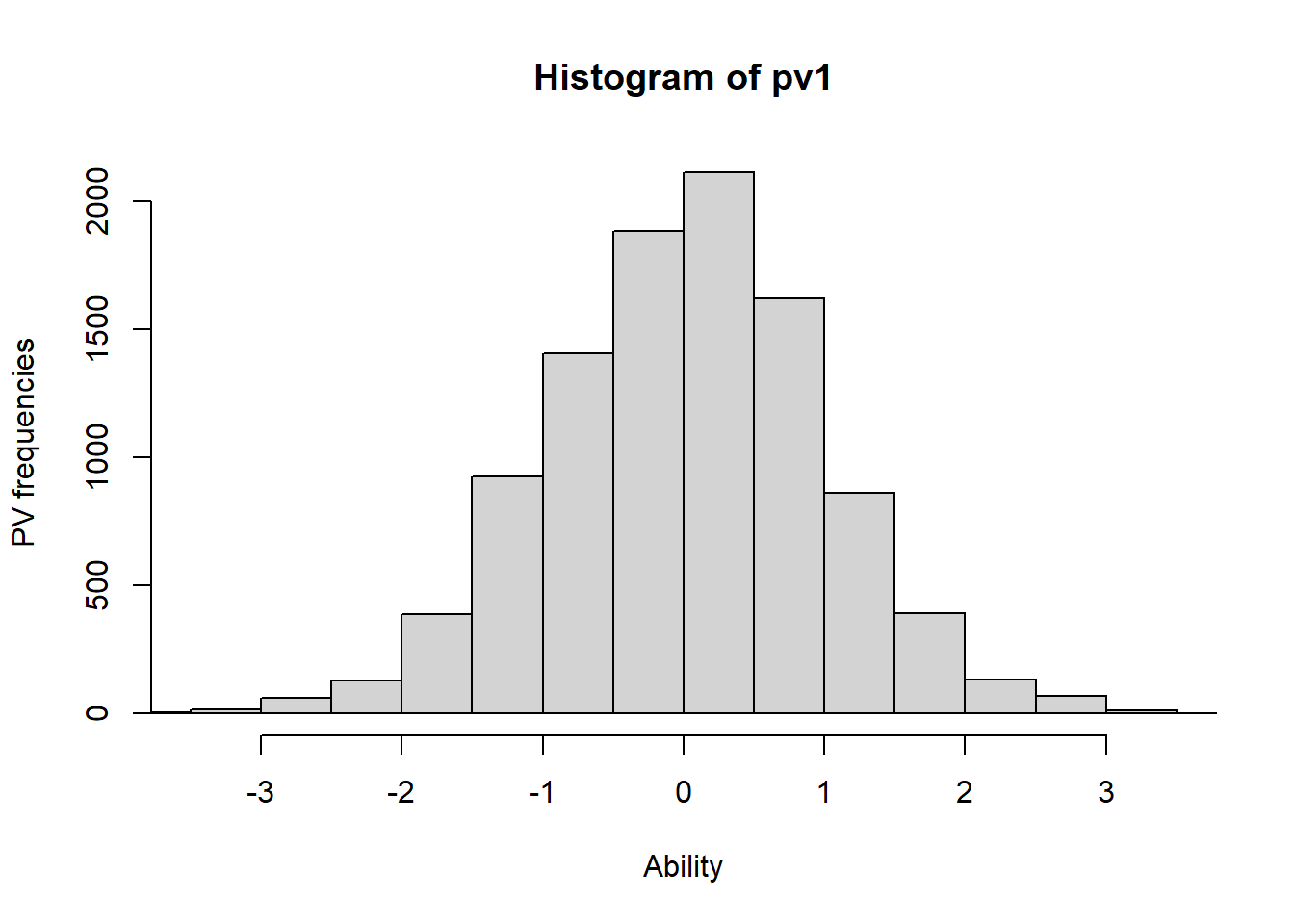Figure 14.6: PV ability distribution For WLE and EAP ability estimates, students with the same test score have exactly the same ability estimate. So if there are 10 items, there are 11 possible scores (0 to 10), and 11 distinct WLE ability estimates and 11 EAP ability estimates. When the ability distribution is discrete, there is a problem with calculating percentile points and percentages in levels. Since a percentile point could occur in one group of students with the same ability estimate, or the level cut-points could happen in between two ability estimates so that the cut-points can be anywhere between two ability estimates and the number of students in the levels remain the same. Further, we can see in Figures 14.4, 14.5 and 14.6 that the WLE distribution is wider, and EAP distribution is narrower than the PV distribution, reflecting the over-estimate and under-estimate of the variance respectively. ## 14.10 How to use plausible values The simplest way to use plausible values is to choose one set (e.g, the first PV for every student) and compute the statistics you want, for example, the mean score. In our example above, we just chose the first PV, and computed mean and variance. We found that these matched the generating (true) values well. Of course, you can choose the second set of PVs, or the third set, etc. Each time you will get slightly different estimates. To combine results from multiple sets of PVs, simply take the average of the estimates. Note that this is different from first taking the average for each student, and then compute the statistic. Let’s do an example. The following R code assumes that PVs have already been drawn. pv10 <- pv$pv[,-1]  #Remove 1st column which is person ID.
TenMean <- apply(pv10,2,mean)  #Calculate mean for each set of PV
mean(TenMean)
TenVar <- apply(pv10,2,var)  #Calculate variance for each set of PV
mean(TenVar)

The above procedure is simple. However, when it comes to computing the standard errors of the statistics, the procedure becomes a little more complex, because we need to combine both sampling error and measurement error.

## 14.11 Computing statistics and standard errors using plausible values

The procedure of using PVs to estimate a population statistic is as follows:

1. Calculate the statistic, $$T$$, using each set of PV. If there are D sets of PVs, we will have $$T_1, T_2, ..., T_D$$.
2. Calculate the average of the $$T_i$$:

$$T=\frac{1}{D} \sum\limits_{i=1}^D T_i$$
This is the estimate of the population statistic, $$T$$.

The procedure of using PVs to estimate the standard error of a statistic is as follows:

1. Calculate the measurement variance:

$$v_m=\frac{1}{D-1} \sum\limits_{i=1}^D (T_i-T)^2$$

1. Calculate sampling variance, $$v_s$$.

If the sample of student is a simple random sample, there are usually formulas for calculating the sampling variance. If the sampling design is complex, then replication methods will be needed. In PISA, balanced repeated replicates are used for calculating the sampling variance, while TIMSS uses a Jacknife approach which also involves replications.

1. Combine measurement variance and sampling variance using the formula below:

$\begin{equation} v = v_s + (1+\frac{1}{D})v_m\tag{14.1} \end{equation}$

1. The standard error of the statistic, $$T$$, is

$$se(T) = \sqrt{v}$$

## 14.12 An Example

We will use the estimation of population mean as an example.

The following R code assumes that an item response data set called resp has been generated.

mod1 <- tam.mml(resp)
pv <- tam.pv(mod1)  #Draw plausible values. By default, 10 PVs are drawn for each student.
pv10 <- pv\$pv[,-1]  #Remove 1st column which is person ID.
TenMean <- apply(pv10,2,mean)  #Calculate mean for each set of PV
T <- mean(TenMean)  # This is our estimate for the population mean

# Measurement variance
v_m <- var(TenMean)

# Sampling variance for mean of simple random sample
TenVar <- apply(pv10,2,var)/nrow(pv10)
# If complex sampling is used, TenVar will be computed using replication method
v_s <- mean(TenVar)
v <- v_s + (1+1/ncol(pv10))*v_m
se <- sqrt(v)

## 14.13 Summary of analysis procedures

The process of MML estimation and the production and use of plausible values are illustrated below:

1. Collect item response data

2. MML scaling to estimate ability distribution parameters (Prior)

We should be able to stop here. However, to enable secondary data analysis, we need to provide data that can be processed using standard statistical software.

1. Compute posterior distribution for each student

2. Draw PV for each student.

3. Distribute data sets of plausible values and other variables.

When secondary data analysts analyse the data, the process is reversed.

1. Aggregate plausible values to form population statistics. The plausible values will reconstruct posterior distributions which will in turn aggregate to the prior ability distribution or a subgroup of the ability distribution.

2. Compute standard errors of estimates, taking into account of both the measurement and sampling errors.

## 14.14 Homework

Simulate an item response data set with 5000 students and 20 items. Scale the data with both the JML and MML estimation methods. Answer the following questions:

1. What are the direct estimates of population mean and variance from the MML scaling?

2. What are the estimates of population mean and variance computed from WLE produced by the JML scaling?

3. What are the estimates of population mean and variance computed from EAP produced by the MML scaling?